## Remove text within parentheses or brackets from text strings

Supposing, you have a list of text strings, and part of the texts are enclosed in the parentheses, now, you want to remove all texts within the parentheses and including the parentheses themselves as below screenshot shown. This article, I will introduce some methods for solving this task in Excel.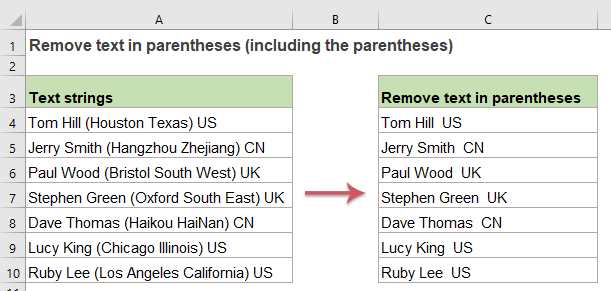#### Remove text within parentheses or brackets from text strings with formula

You can create a formula based on the SUBSTITUTE, MID FIND and LEN functions for dealing with this job, the generic syntax is:

=SUBSTITUTE(text,MID(LEFT(text,FIND(")",text)),FIND("(",text),LEN(text)),"")
• text: The text string or cell reference that you want to use.

Please copy or enter the following formula into a blank cell where you want to get the result:

=SUBSTITUTE(A2,MID(LEFT(A2,FIND(")",A2)),FIND("(",A2),LEN(A2)),"")

And then, drag the fill handle down to the cells you want to apply this formula, and all texts within the parentheses including the parentheses have been removed, see screenshot: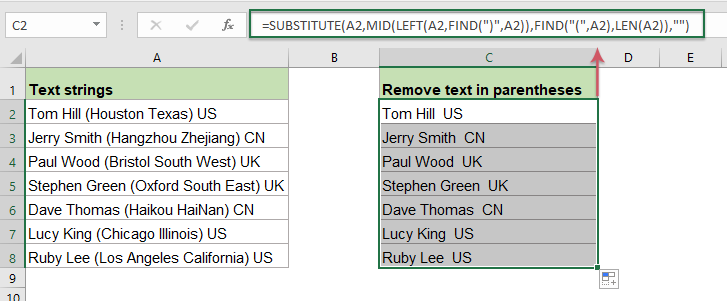##### Explanation of the formula:

1. MID(LEFT(A2,FIND(")",A2)),FIND("(",A2),LEN(A2)): This MID function is recognized as the old_text argument within the SUBSTITUTE function.

• LEFT(A2,FIND(")",A2)): This part of the formula is used to extract the text string from left to the right parenthesis in cell A2, and you will get the result as this: “Tom Hill (Houston Texas)”. This will be recognized as the text argument within the MID function.
• FIND("(",A2): This FIND function will return the position of the left parenthesis from cell A2, the result is: 10. And this part formula is recognized as the start_num argument within the MID function.
• LEN(A2): The LEN function will get the total number of the characters in cell A2, and the result is: 27. This part is recognized as the num_chars argument of the MID function.
• MID(LEFT(A2,FIND(")",A2)),FIND("(",A2),LEN(A2))= MID("Tom Hill (Houston Texas)",10,27): This MID function is used to extract the characters from the text string which are returned by the LEFT function, start from the tenth character with a length of 27 characters, and you will get the result as this: “(Houston Texas)”.

2. SUBSTITUTE(A2,MID(LEFT(A2,FIND(")",A2)),FIND("(",A2),LEN(A2)),"")= SUBSTITUTE(A2, "(Houston Texas)",""): At last, this SUBSTITUTE function is used to replace the old text that returned by the MID function with nothing in the text string of cell A2.

##### Notes:

1. If the part of text enclosed with the brackets, you just need to replace the parentheses with the brackets as below formula:

=SUBSTITUTE(A2,MID(LEFT(A2,FIND("]",A2)),FIND("[",A2),LEN(A2)),"")

2. If there is no parentheses in the cell value, after applying the above formula, an error will be displayed, in this case, you just need to enclose the above formula into the IFERROR function:

=IFERROR(SUBSTITUTE(A2,MID(LEFT(A2,FIND(")",A2)),FIND("(",A2),LEN(A2)),""),A2)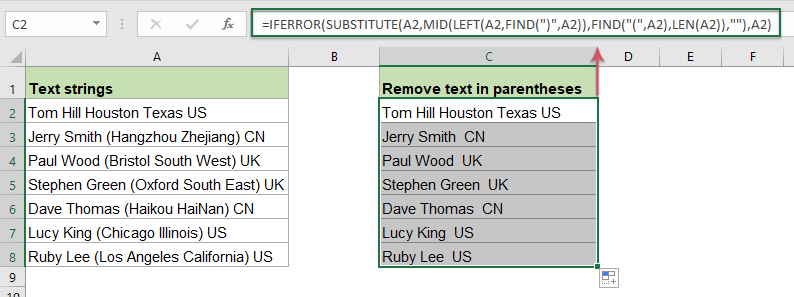#### Remove text within parentheses or brackets from text strings with User Defined Function

If there are two or more parentheses within the text string, the above formula only can be used to remove text in the first parentheses. To remove all texts in multiple parentheses as the following screenshot shown, how could you achieve it in Excel?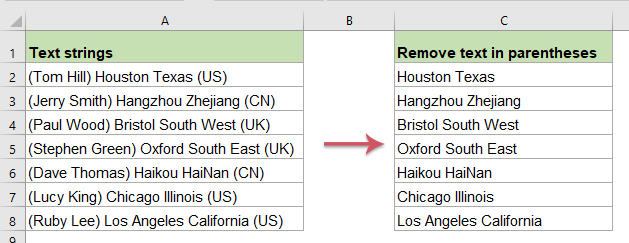In this case, a User Defined Function can help you to remove all texts in the parentheses. Please do with the following steps:

1. Hold down the Alt + F11 keys in Excel, and it opens the Microsoft Visual Basic for Applications window.

2. Click Insert > Module, and paste the following VBA code in the Module Window.

``````Function remtxt(ByVal str As String) As String
'updateby Extendoffice
While InStr(str, "(") > 0 And InStr(str, ")") > InStr(str, "(")
str = Left(str, InStr(str, "(") - 1) & Mid(str, InStr(str, ")") + 1)
Wend
remtxt = Trim(str)
End Function
``````

3. Then, go back to the worksheet where you want to use, and enter this formula into a blank cell: =remtxt(A2), then drag the fill handle down to the cells you want to apply this formula, all the texts within the multiple parentheses including the parentheses have been removed at once, see screenshot: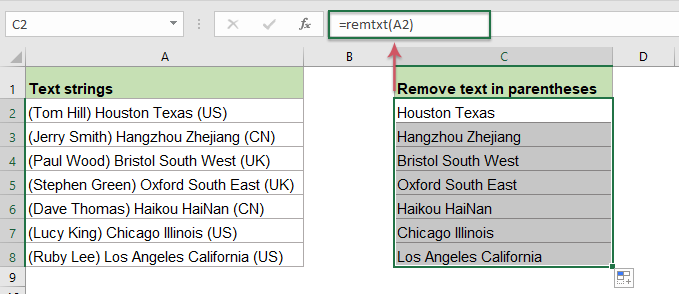#### Relative functions used:

• LEN:
• The LEN function returns the number of characters in a text string.
• MID:
• The MID function is used to find and return a specific number of characters from the middle of given text string.
• FIND:
• The FIND function is used to find a string within another string, and returns the starting position of the string inside another one.
• SUBSTITUTE:
• The Excel SUBSTITUTE function replaces text or characters within a text string with another text or characters.

### The Best Office Productivity Tools

#### Kutools for Excel - Helps You To Stand Out From Crowd

 Popular Features: Find, Highlight or Identify Duplicates  |  Delete Blank Rows  |  Combine Columns or Cells without Losing Data  |  Round without Formula ... Super VLookup: Multiple Criteria  |  Multiple Value  |  Across Multi-Sheets  |  Fuzzy Lookup... Adv. Drop-down List: Easy Drop Down List  |  Dependent Drop Down List  |  Multi-select Drop Down List... Column Manager: Add a Specific Number of Columns  |  Move Columns  |  Toggle Visibility Status of Hidden Columns  |  Compare Columns to Select Same & Different Cells ... Featured Features: Grid Focus  |  Design View  |  Big Formula Bar  |  Workbook & Sheet Manager | Resource Library (Auto Text)  |  Date Picker  |  Combine Worksheets  |  Encrypt/Decrypt Cells  |  Send Emails by List  |  Super Filter  |  Special Filter (filter bold/italic/strikethrough...) ... Top 15 Toolsets:  12 Text Tools (Add Text, Remove Characters ...)  |  50+ Chart Types (Gantt Chart ...)  |  40+ Practical Formulas (Calculate age based on birthday ...)  |  19 Insertion Tools (Insert QR Code, Insert Picture from Path ...)  |  12 Conversion Tools (Numbers to Words, Currency Conversion ...)  |  7 Merge & Split Tools (Advanced Combine Rows, Split Excel Cells ...)  |  ... and more

Kutools for Excel Boasts Over 300 Features, Ensuring That What You Need is Just A Click Away...#### Office Tab - Enable Tabbed Reading and Editing in Microsoft Office (include Excel)

• One second to switch between dozens of open documents!
• Reduce hundreds of mouse clicks for you every day, say goodbye to mouse hand.
• Increases your productivity by 50% when viewing and editing multiple documents.
• Brings Efficient Tabs to Office (include Excel), Just Like Chrome, Edge and Firefox.No ratings yet. Be the first to rate!
This comment was minimized by the moderator on the site
J'ai testé cette formule mais elle ne fonctionne pas sur GGsheet
This comment was minimized by the moderator on the site
Hello, Camille
To remove text within parentheses in Google Sheets, please apply the below formula:
``=regexreplace(A2, "(\s\(.*?\))",)``